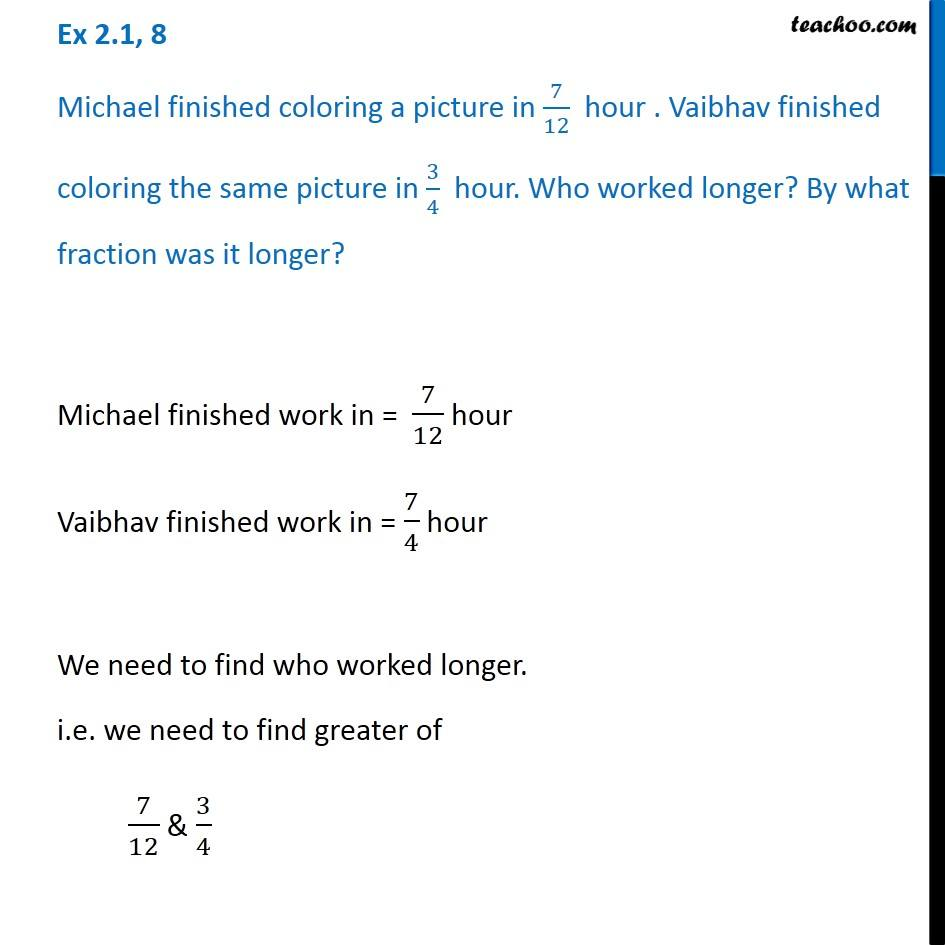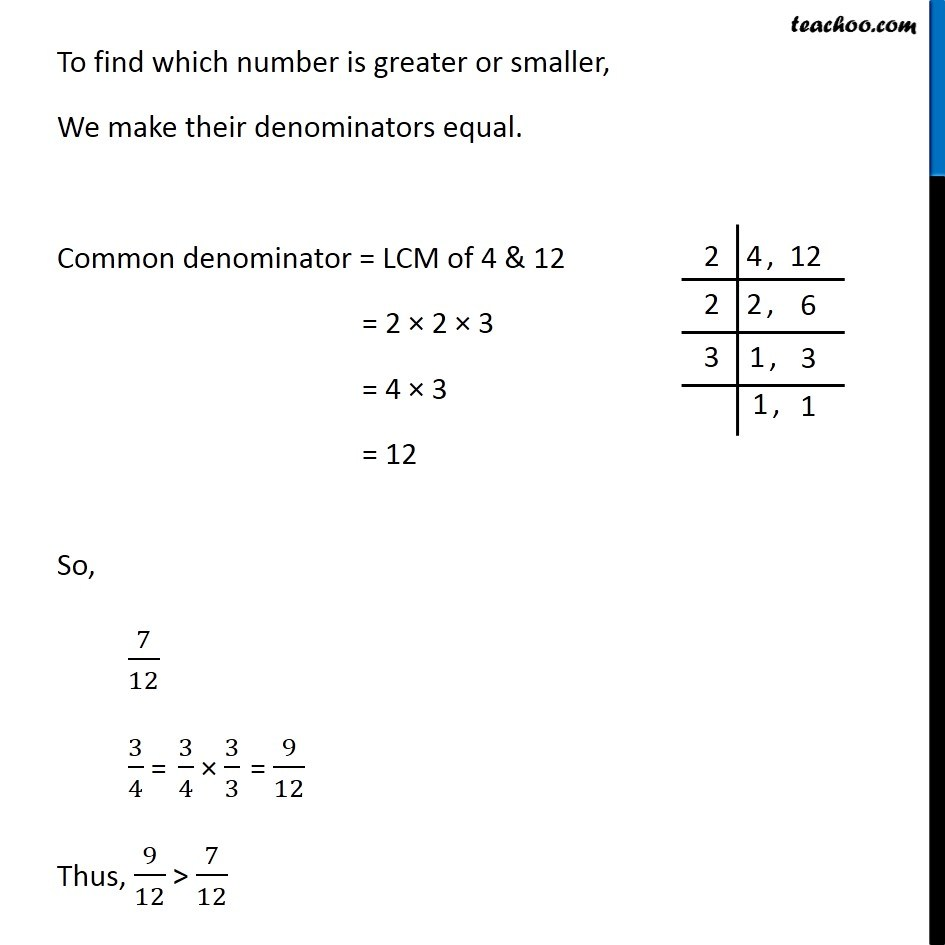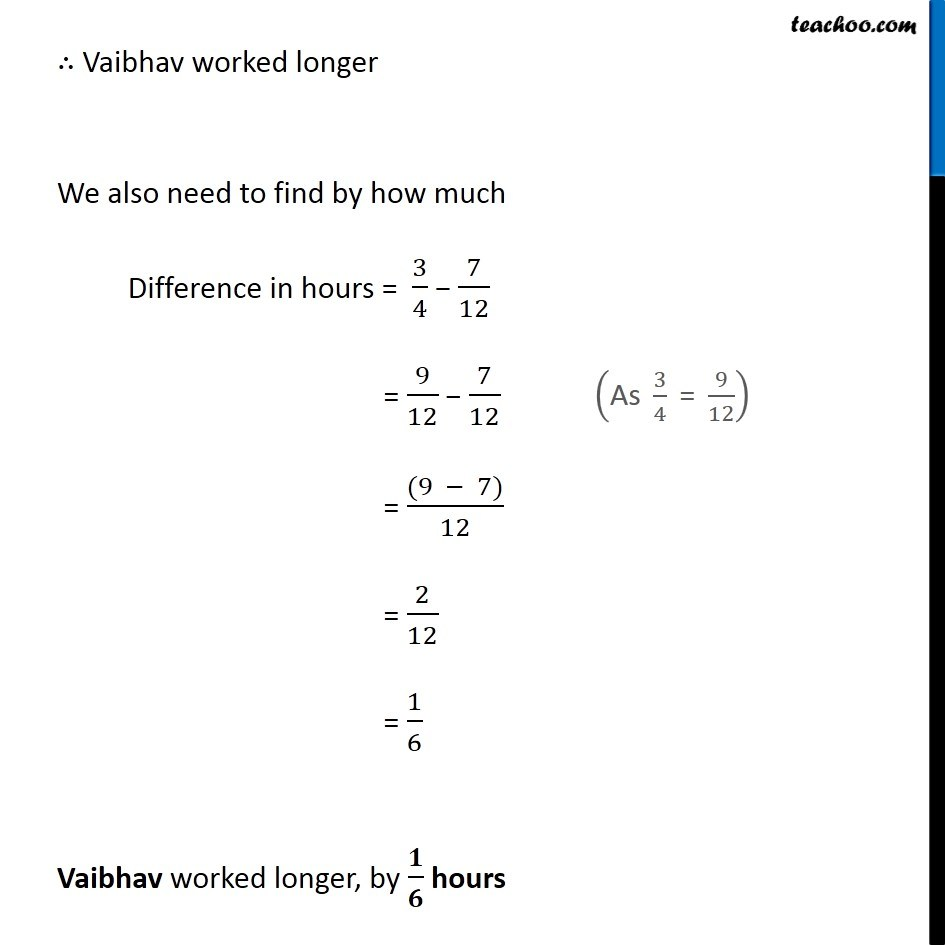1. Chapter 2 Class 7 Fractions and Decimals
2. Concept wise
3. Greater than, less than in fractions

Transcript

Ex 2.1, 8 Michael finished coloring a picture in 7/12 hour . Vaibhav finished coloring the same picture in 3/4 hour. Who worked longer? By what fraction was it longer? Michael finished work in = 7/12 hour Vaibhav finished work in = 7/4 hour We need to find who worked longer. i.e. we need to find greater of 7/12 & 3/4 To find which number is greater or smaller, We make their denominators equal. Common denominator = LCM of 4 & 12 = 2 × 2 × 3 = 4 × 3 = 12 So, 7/12 3/4 = Thus, 9/12 > 7/12 ∴ Vaibhav worked longer We also need to find by how much Difference in hours = 3/4 − 7/12 = 9/12 − 7/12 = ((9 − 7))/12 = 2/12 = 1/6 Vaibhav worked longer, by 𝟏/𝟔 hours

Greater than, less than in fractions

Chapter 2 Class 7 Fractions and Decimals
Concept wise